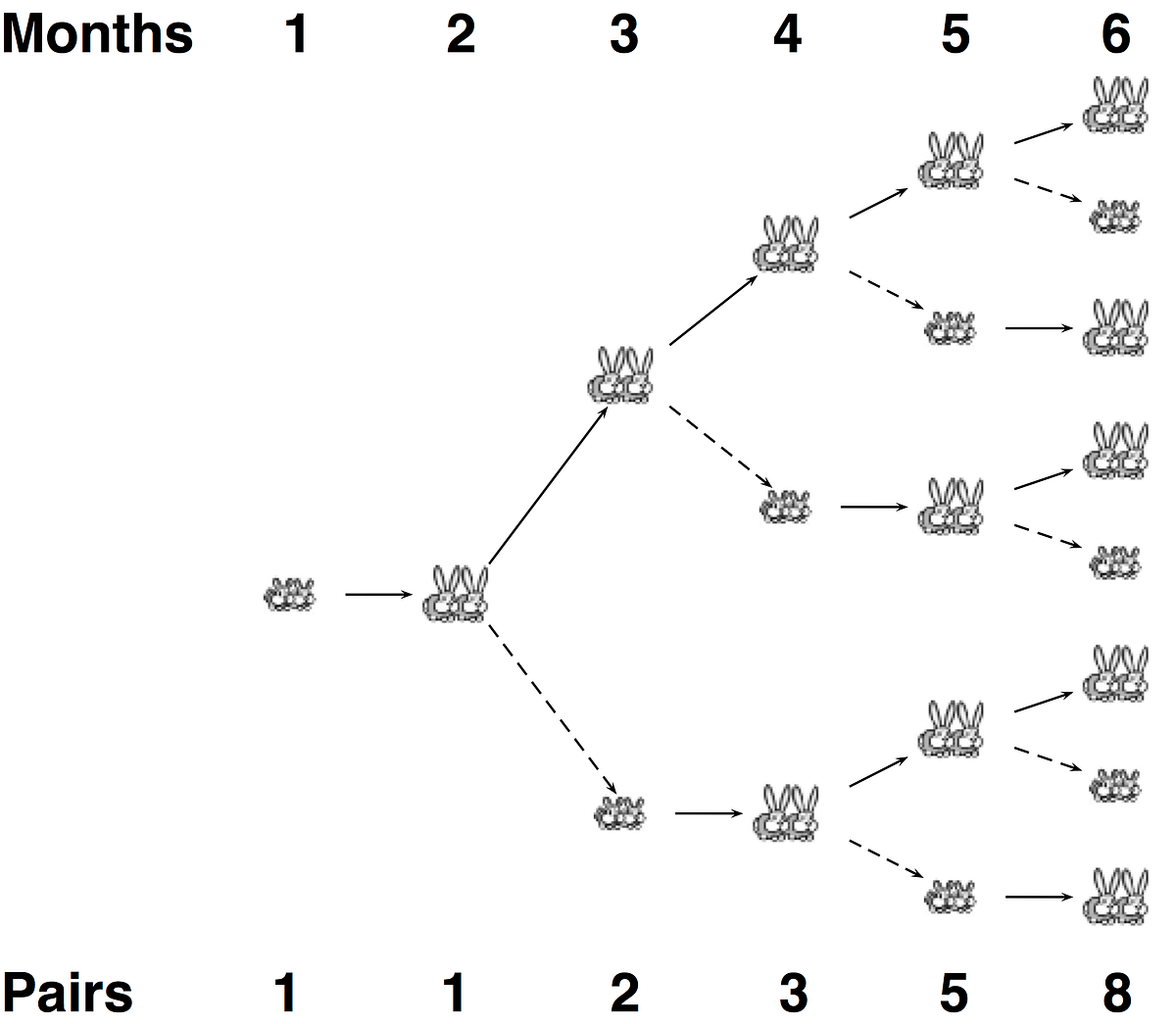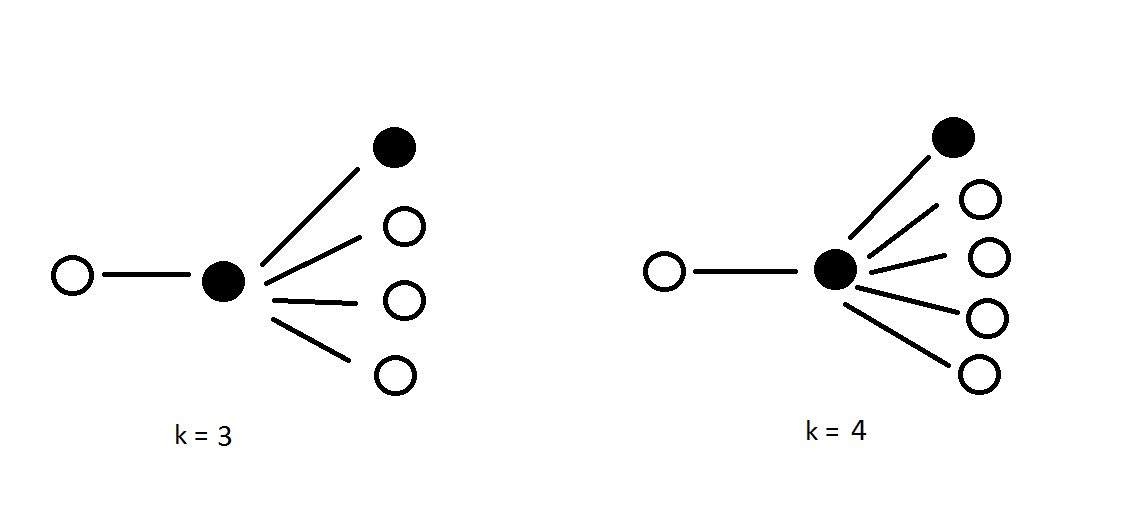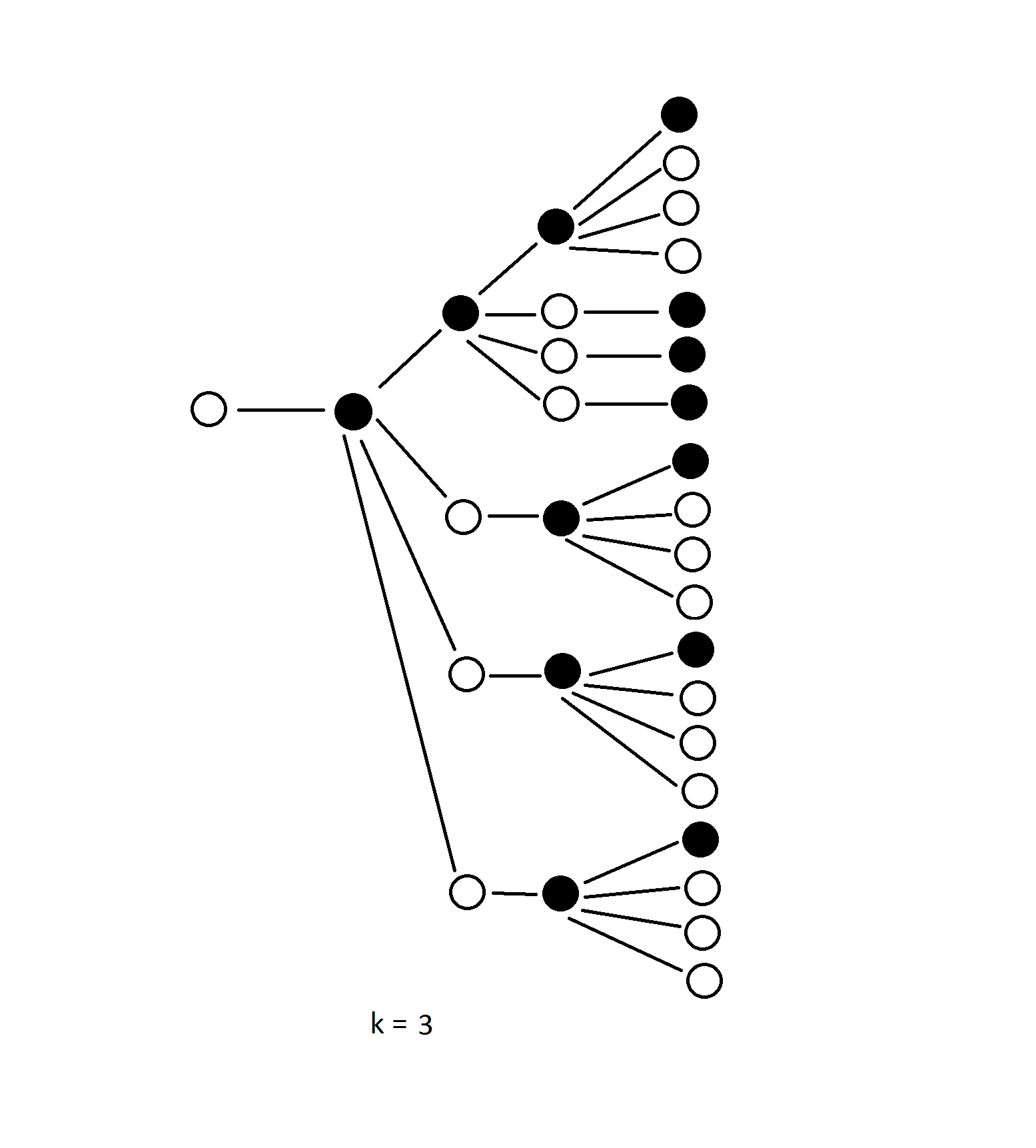# ROSALIND WALKTHROUGH: Rabbits and Recurrence Relations

I’d like to start out by saying this question was a pain in the ass to solve. Partly because I didn’t read the question properly and partly because I wasn’t paying attention in class, 2 months ago, when data types were covered. On that happy note let’s solve today’s question.

A sequence is an ordered collection of objects (usually numbers), which are allowed to repeat. Sequences can be finite or infinite. Two examples are the finite sequence (π,−2√,0,π) and the infinite sequence of odd numbers (1,3,5,7,9,…). We use the notation an to represent the n-th term of a sequence.
A recurrence relation is a way of defining the terms of a sequence with respect to the values of previous terms. In the case of Fibonacci’s rabbits from the introduction, any given month will contain the rabbits that were alive the previous month, plus any new offspring. A key observation is that the number of offspring in any month is equal to the number of rabbits that were alive two months prior. As a result, if Fn represents the number of rabbit pairs alive after the n-th month, then we obtain the Fibonacci sequence having terms Fn that are defined by the recurrence relation Fn=Fn−1+Fn−2 (with F1=F2=1 to initiate the sequence). Although the sequence bears Fibonacci’s name, it was known to Indian mathematicians over two millennia ago.
When finding the n-th term of a sequence defined by a recurrence relation, we can simply use the recurrence relation to generate terms for progressively larger values of n. This problem introduces us to the computational technique of dynamic programming, which successively builds up solutions by using the answers to smaller cases.
Given: Positive integers n≤40 and k≤5.
Return: The total number of rabbit pairs that will be present after n months if we begin with 1 pair and in each generation, every pair of reproduction-age rabbits produces a litter of k rabbit pairs (instead of only 1 pair).

There’s a lot to take in here in order to answer this question but lets break it down into steps. The first thing that is mentioned is recurrence relations. As the introduction states, a recurrence relation defines the terms of a sequence with respect to the values of previous terms. Let’s apply this to a population of rabbits.Rabbit population growth for the first 6 months

There are two things to make note of here. First is the fact that there are small rabbits — which represent offspring and large rabbits which represent the adults. The key point here is that it takes 1 month for the offspring to become adults.

The second point is that the population for each month is determined by the months before it. For example, in the fifth month there are five rabbits. Because the population grows as a Fibonacci sequence, by adding the number of rabbits in the fourth and third generations together, we get the total number of rabbits in the current generation.

Having covered that, let’s get into the code.

`long rabbitPairs(int numMonths, int numOffspring) {`
`    if (numMonths == 1) {`
`        return 1;    }`
`    else if (numMonths == 2) {`
`        return numOffspring;    }`
`    long oneGen = rabbitPairs(numMonths — 1, numOffspring);    long twoGen = rabbitPairs(numMonths — 2, numOffspring);`
`    if (numMonths <= 4) {`
`        return (oneGen + twoGen);    }`
`    return (oneGen + (twoGen * numOffspring));}`

At this point your probably thinking that this little bit of code was definitely not worth the long introduction. Bear with me though since there is a lot going on conceptually.

I started by defining a function called rabbitPairs that took the number of months as well as the number of offspring as parameters. This function returns a long integer. We’ll get to why I chose long instead of int as the return type later on.

With a recursive function you always start by defining a base case. Since this is a function that calls itself it needs to have some way to stop. From the intro we know that during the first month there is only one pair of rabbits so I defined a condition for that.

`if (numMonths == 1) {`
`    return 1;}`

Now in the case of the second and third month, the number of rabbit’s follows a different pattern. Let’s look at some examples I came up with.Rabbit populations for the first 3 months when k = 3 and k = 4

As you can see the number of rabbits in the third month is the number of offspring (white) per mating cycle plus one adult (black). Therefore the condition for the second month would be

`else if (numMonths == 2) {`
`    return numOffspring;}`

since adding the values of the second month and first month together will give us the correct value for the third month.

Now that the base cases are defined the next step is to keep calling our function until it reaches one of the base cases. We know that Fibonacci’s sequence is defined as F(n) = F(n-1) + F(n-2) so we use

`long oneGen = rabbitPairs(numMonths — 1, numOffspring);long twoGen = rabbitPairs(numMonths — 2, numOffspring);`

to keep calling our function until the base cases are reached.

Finally, we get to the last bit of the code.

`if (numMonths <= 4) {`
`    return (oneGen + twoGen);}`
`return (oneGen + (twoGen * numOffspring));`

where you’ll notice the condition

`if (numMonths <= 4) {`
`    return (oneGen + twoGen);}`

The reason for this, is that up until the fourth generation the size of the population can be predicted by adding up the rabbit populations one and two generations ago. After four months however,

`return (oneGen + (twoGen * numOffspring));`

is used to calculate the number of rabbits. Why? Let’s take a look at an example.Rabbit population after 5 months when k = 3

We can see that the number of rabbits two generations back is equal to the number of adults one generation back. As an example, there are four rabbits total in the third generation which results in four adults in the fourth generation. Multiplying the number of offspring born during each mating with the number of rabbits two generations ago, gives us the number of offspring in the current generation. We simply add this to the number of rabbits one generation ago to get our answer.

Whew! Well let’s run our code and test it out.

Number of months: 29

Number of Offspring: 2

`long rabbitPairs(int numMonths, int numOffspring) {`